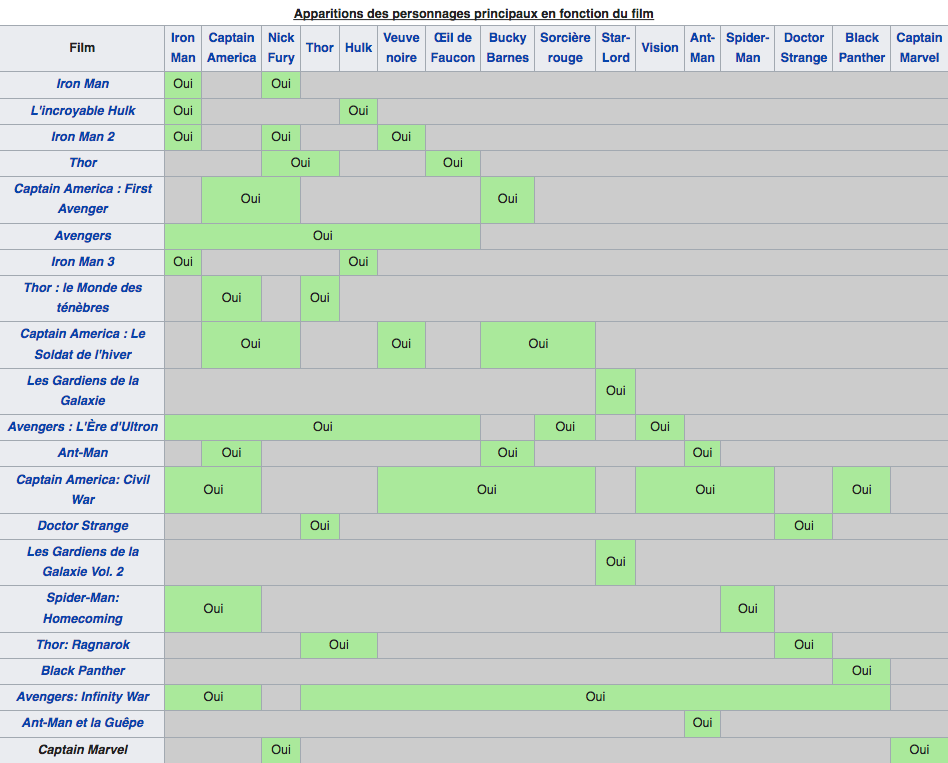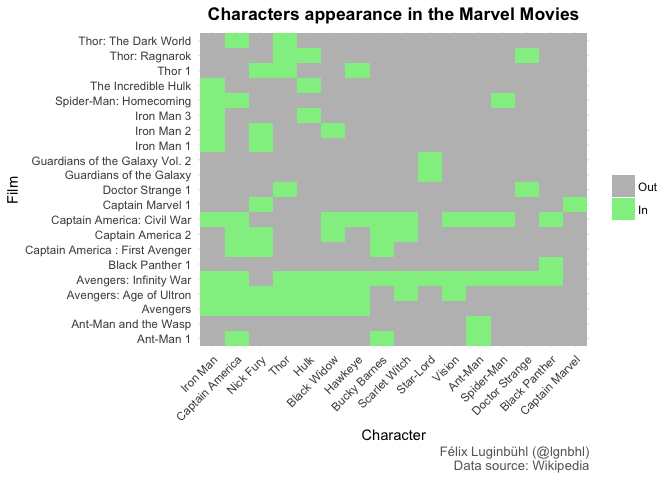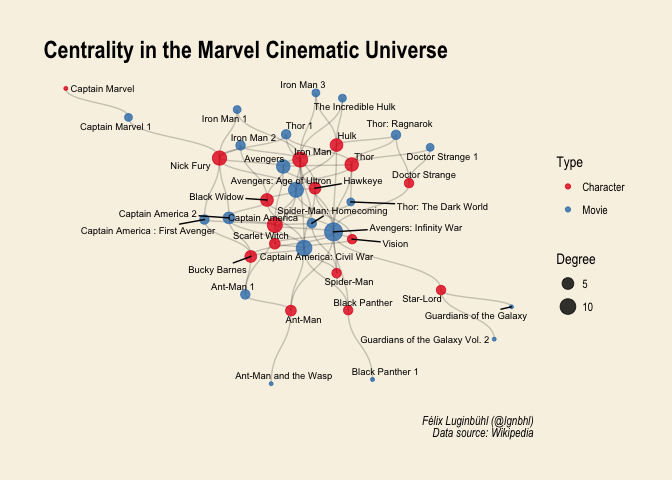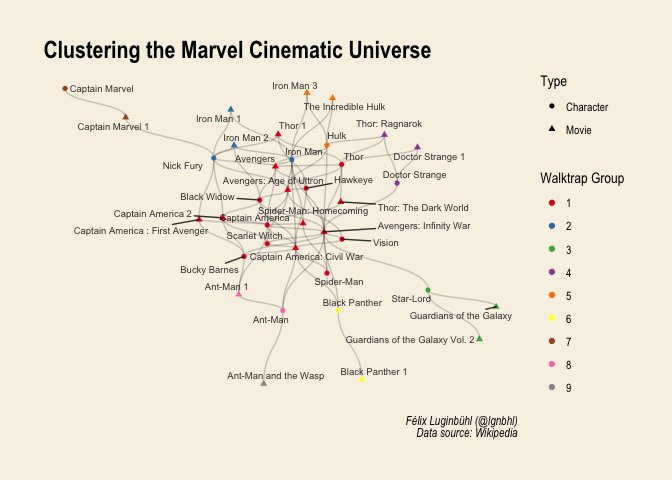# Which Marvel Characters and Movies are the Most Central?

To begin this year, I was looking for a quick project related to social network visualization. In this blog post, we will find out which characters and movies are the most central in the Marvel cinematic universe.

As R analyses on Game of Thrones, Star Wars or The Lord of The Rings have already been done, I decided to visualize the superhero network of the nowadays very popular Marvel movies saga.

## Scraping Marvel superheros

As often, the data can be found on Wikipedia. But surprisingly, the data needed was not avaiable in a English article, but within this French article.Let’s scrape this wikitable with the `rvest` package.

``````library(tidyverse)
library(rvest)
url <- "https://fr.wikipedia.org/w/index.php?title=Liste_des_films_de_l%27univers_cin%C3%A9matographique_Marvel&oldid=144793972#Personnages"

marvel_df <- url %>%
html_nodes(".wikitable") %>%
html_table(fill = TRUE) %>%
.[]``````

Now that we have the data, we need to clean it as well as translate some names in English. We also have to distinguish the movie names from the characters.

``````marvel_df[1,1] <- "Iron Man 1"
marvel_df[2,1] <- "The Incredible Hulk"
marvel_df[4,1] <- "Thor 1"
marvel_df[8,1] <- "Thor: The Dark World"
marvel_df[9,1] <- "Captain America 2"
marvel_df[10,1] <- "Guardians of the Galaxy"
marvel_df[11,1] <- "Avengers: Age of Ultron"
marvel_df[12,1] <- "Ant-Man 1"
marvel_df[14,1] <- "Doctor Strange 1"
marvel_df[15,1] <- "Guardians of the Galaxy Vol. 2"
marvel_df[18,1] <- "Black Panther 1"
marvel_df[20,1] <- "Ant-Man and the Wasp"
marvel_df[21,1] <- "Captain Marvel 1"

marvel_df <- marvel_df %>%
rename("Black Widow" = "Veuve noire",
"Hawkeye" = "Œil de Faucon",
"Scarlet Witch" = "Sorcière rouge") %>%
mutate(Film = factor(Film, levels = unique(Film))) %>%
mutate_all(funs(str_replace_all(., c("Oui" = "1", "^\$" = "0")))) #^\$ is for empty string``````

## Reproducing the wikitable with a heatmap

Let’s tidy the data in order to reproduce the Wikipedia table with `ggplot2`. Note that the characters and movies are in a different order.

``````marvel_tidy <- marvel_df %>%
reshape2::melt(id.vars = "Film", value.name = "Value") %>%
rename("Character" = "variable")

ggplot(marvel_tidy, aes(x = Character, y = Film)) +
geom_tile(aes(fill = Value)) +
scale_fill_manual(values=c("0"="grey", "1"="lightgreen"),
name="", labels=c("Out","In")) +
theme_minimal() +
theme(plot.title = element_text(face = "bold", hjust = 0.5),
plot.caption = element_text(colour = "dimgrey"),
axis.text.x = element_text(angle = 45, vjust = 1, hjust=1)) +
labs(title = "Characters appearance in the Marvel Movies",
caption = "Félix Luginbühl (@lgnbhl)\nData source: Wikipedia")``````## Centrality metrics

In order to know which characters and movies are the most central, we can use indicators like the degree (number of ties) and the closeness (centrality based on distance to others in the graph).

``````library(tidygraph)

marvel_graph <- marvel_tidy %>%
filter(Value != "0") %>%
select(-Value) %>%
as_tbl_graph(directed = FALSE) %>%
mutate(degree = centrality_degree(),
closeness = centrality_closeness(),
betweenness = centrality_betweenness()) %>%
#create type variable
full_join(tibble(film = marvel_df\$Film, type = "Movie"), by = c("name" = "film")) %>%
mutate(type = replace_na(type, "Character"))

marvel_graph %>%
activate(nodes) %>%
as_tibble() %>%
arrange(desc(degree))``````
``````## # A tibble: 36 x 5
##    name                       degree closeness betweenness type
##    <chr>                       <dbl>     <dbl>       <dbl> <chr>
##  1 Avengers: Infinity War        14.    0.0161       237.  Movie
##  2 Captain America: Civil War    10.    0.0128        57.8 Movie
##  3 Avengers: Age of Ultron        9.    0.0135        61.6 Movie
##  4 Iron Man                       9.    0.0135        89.2 Character
##  5 Captain America                9.    0.0135        69.3 Character
##  6 Nick Fury                      8.    0.0111        94.0 Character
##  7 Avengers                       7.    0.0128        40.7 Movie
##  8 Ant-Man                        7.    0.0123        35.3 Movie
##  9 Thor                           7.    0.0128        62.2 Character
## 10 Hulk                           6.    0.0125        40.1 Character
## # ... with 26 more rows``````

The central characters are Iron Man and Captain America (ex aequo), followed by Nick Fury, Thor and Hulk. The central movies are the two Avengers movies and as well as Captain America: Civil War (2016).

Who is the most distant character from Iron Man?

``````marvel_graph %>%
mutate(distance = bfs_dist(name == "Iron Man", mode = "all")) %>%
filter(type == "Character") %>%
select(name, distance) %>%
arrange(desc(distance))``````
``````## # A tbl_graph: 15 nodes and 0 edges
## #
## # An undirected simple graph with 15 components
## #
## # Node Data: 15 x 2 (active)
##   name            distance
##   <chr>              <int>
## 1 Captain Marvel         4
## 2 Captain America        2
## 3 Nick Fury              2
## 4 Thor                   2
## 5 Hulk                   2
## 6 Black Widow            2
## # ... with 9 more rows
## #
## # Edge Data: 0 x 2
## # ... with 2 variables: from <int>, to <int>``````

Captain Marvel is the most distant character. However, as the movie introducing Captain Marvel will only be released in 2019, other Marvel characters could be added to the movie (and therefore reducing the distance from Iron Man).

## Plotting the network

Now let’s visualize the centrality degree of the movies and characters using {ggraph}.

``````library(ggraph)
set.seed(100)

ggraph(marvel_graph, layout = "nicely") +
geom_edge_diagonal(alpha = 0.2) +
geom_node_point(aes(size = degree, color = as.factor(type)), alpha = 0.8) +
scale_color_brewer(palette = "Set1", name = "Type") +
geom_node_text(aes(label = name), size = 2.5, repel = TRUE) +
theme_graph() +
theme(plot.background = element_rect(fill = "#f8f2e4")) +
labs(title = "Centrality in the Marvel Cinematic Universe",
size = "Degree",
caption = "Félix Luginbühl (@lgnbhl)\n Data source: Wikipedia")``````Now let’s try some clustering, with the Walktrap algorithm.

``````set.seed(100)

marvel_graph %>%
activate(nodes) %>%
mutate(group_walktrap = group_walktrap()) %>%
ggraph(layout = "nicely") +
geom_edge_diagonal(alpha = 0.2) +
geom_node_point(aes(color = as.factor(group_walktrap), shape = as.factor(type))) +
geom_node_text(aes(label = name), size = 2.5, alpha = 0.8, repel = TRUE) +
scale_color_brewer(palette = "Set1", name = "Walktrap Group") +
theme_graph() +
theme(plot.background = element_rect(fill = "#f8f2e4")) +
labs(title = "Clustering the Marvel Cinematic Universe",
shape = "Type",
caption = "Félix Luginbühl (@lgnbhl)\n Data source: Wikipedia")``````The walktrap algorithm is doing a good job, as the characters and the movies seem correctly grouped.

## An interactive visualization of the Marvel Universe Network

Making an interactive network with {visNetwork} is quite easy. Just play with the interactive network below.

``````library(visNetwork)

``````